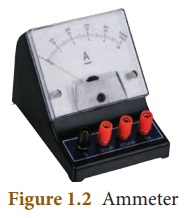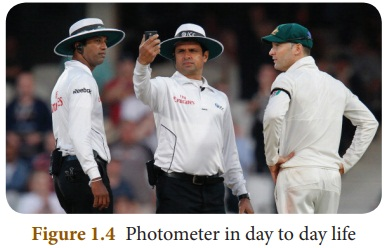Home | | Science 8th Std | International System of Units

# International System of Units

As you studied in the lower classes, in 1960, in the 11th General Conference on Weights and Measures at Paris in France, scientists recognised the need of using standard units for physical quantities. That was called as ‘International System of Units’ and is popularly known as SI System (abbreviated from the French name ‘Systeme International’).

International System of Units

In earlier days, scientists performed their experiments and recorded their results in their own system. Due to lack of communication, they couldn’t organise experimental results of others. So, they planned to follow a uniform system for taking the measurements.

As you studied in the lower classes, in 1960, in the 11th General Conference on Weights and Measures at Paris in France, scientists recognised the need of using standard units for physical quantities. That was called as ‘International System of Units’ and is popularly known as SI System (abbreviated from the French name ‘Systeme International’). Scientists, chose seven physical quantities as ‘Base Quantities’ and defined a ‘Standard Unit’ to measure each one. They are known as Base Units or Fundamental Units (Table 1. 1)You have already studied about length, mass and time in your lower classes. Now you are going to study about the other base quantities such as temperature, current, amount of substance and luminous intensity.

In December, 1998, the National Aeronautics and Space Administration (NASA) USA, launched the Mars Climate Orbiter to collect data about the Martian climate. Nine months later, on September 23, 1999, the Orbiter disappeared while approaching Mars at an unexpectedly low altitude. An investigation revealed that the orbital calculations were incorrect due to an error in the transfer of information between the spacecraft’s team in Colorado and the mission navigation team in California. One team was using the English FPS system of units for calculation, while the other team was using the MKS system of units. This misunderstanding caused a loss of 125 million dollars approximately.

1. Temperature

Identify, which of the following objects are hot and which of them are cold?We see a number of objects in our daily life. Some of them are cold and some of them are hot. Some times we may say that two objects are equally hot or cold. But, there will be some difference in their hotness or coldness. How do you decide, which is hotter and which is colder? You need a reliable quantity to decide the degree of hotness or coldness of an object. That quantity is ‘temperature’.

Temperature is a physical quantity that expresses the degree of hotness or coldness of a substance. Heat energy given to a substance will increase its temperature. Heat energy removed from a substance will lower its temperature.

Temperature is defined as a measure of the average kinetic energy of the particles in a system. The SI unit of temperature is kelvin. Thermometers are used to measure the temperature directly. Usually, thermometers are calibrated with some standard scales. Celsius, Fahrenheit, Kelvin are the most commonly used scales to measure temperature.

ACTIVITY

Measure the room temperature inside the class room and outside the class room by using a thermometer and tabulate it with different time intervals for a week. Do you find any differences in these values? Discuss your observations.

Aim : To measure the room temperature inside the class room and outside the class room at different time intervals for a week.

Materials required : Thermometer.

Procedure :

(i) With the help of a thermometer, note down the room temperature inside and outside the class room at different time intervals of a day.

(ii) Repeat the process for 5 days.

(iii) Note down the temperature in the tabular column.Inference:

(i) Temperature varies at different time intervals.

(ii) It varies from place to place.

Activity 2

From the news paper or television, collect the highest and lowest temperature experienced in your nearest town or city for a week and record the values in a tabular column. Does this data remain same throughout the year?Inference: The data (temperature) does not remain same throughout the week.

2. Electric Current (I)

Flow of electric charges, in a particular direction is known as ‘electric current’. The magnitude of electric current is the amount of electric charges flowing through a conductor in one second.

Electric current = Amount of electric charge / timeI = Q / t

Electric charge is measured in coulumb. The SI unit of electric current is ampere and it is denoted as A.

If one coulomb of charge is flowing through a conductor in one second, then, the amount of current flowing is said to be one ampere. Ammeter is the device used to measure ‘electric current’ (Fig 1. 2)Activity 3

Connect a battery, an ammeter and a lamp in series as shown in the figure. Note the ammeter reading. It is the amount of current flowing in the circuit.Aim: To measure the current in an electric circuit.

Components required: Battery, Ammeter and Lamp (Bulb).

Procedure :

(i) Connect the battery, ammeter and the lamp in series as shown in the figure.

(iii) It is the current in the circuit.

Inference: Current is measured by using ammeter in an electric circuit.

Problem 1

If 2 coulomb of charge flows through a circuit for 10 seconds, calculate the current.

Solution

Charge (Q) = 2 C; Time (t) = 10 s

I = Q/t = 2/10 = 0.2 A

3. Amount of Substance

Amount of substance is a measure of the number of entities (particles) present in a substance. The entity may be an atom, molecule, ion, electron or proton etc.

Generally, the amount of substance is directly proportional to the number of atoms or molecules.

Can you count the number of copper coins in the picture? We can count them easily. But, can you count the number of copper atoms in a coin? It is very difficult to count the number of atoms because they are not visible. The number of atoms or molecules in a substance is measured in mole. It is a SI uinit.Mole is defined as the amount of substance, which contains 6.023 × 1023 entities. It is denoted as ‘mol’.

More to Know: The number 6.023 × 1023 is also known as Avogadro Number.

4. Luminous IntensityHave you seen these scenes on the television? What is the umpire doing? He is checking the intensity of light by using an instrument. The measure of the power of the emitted light, by a light source in a particular direction, per unit solid angle is called as luminous intensity. The SI unit of luminous intensity is candela and is denoted as ‘cd’.

The light emitted from a common wax candle is approximately equal to one candela. Luminous intensity is measured by ‘photometer’ (Luminous Intensity Meter) which gives the luminous intensity in terms of candela directly.Info bits

Luminous flux or Luminous power is the measure of the perceived power of light. Its SI unit is ‘lumen’.One lumen is defined as the luminous flux of the light produced by the light source that emits one candela of luminous intensity over a solid angle of one steradian.

Apart from the seven fundamental units, we have two more units known as derived units, we will study about them now.

5. Plane angle

Plane angle is the angle made at the intersection of two straight lines or intersection of two planes. The SI unit of plane angle is ‘radian’ and is denoted as ‘rad’Radian is the angle subtended at the centre of a circle by an arc whose length is equal to the radius of the circle (Fig 1. 7).Problem 2

Solution

We know that,

1° = π/180

60° = π/180 × 60 = π/3 radian

Problem 3

Convert 4π into degrees.

Solution

We know that,

π/4 radian = 180/4 = 45°

6. Solid angle

Solid angle is the angle formed by three or more planes intersecting at a common point. It can also be defined as ‘angle formed at the vertex of the cone’. The SI unit of solid angle is ‘steradian’ and is denoted as ‘sr’.

Steradian is the solid angle at the centre of a sphere subtended by a portion whose surface area is equal to the square of the radius of the sphere.Until 1995, plane angle and solid angle were classified under supplementary quantities. In 1995, they were shifted to derived quantities.Tags : Measurement | Chapter 1 | 8th Science , 8th Science : Chapter 1 : Measurement
Study Material, Lecturing Notes, Assignment, Reference, Wiki description explanation, brief detail
8th Science : Chapter 1 : Measurement : International System of Units | Measurement | Chapter 1 | 8th Science